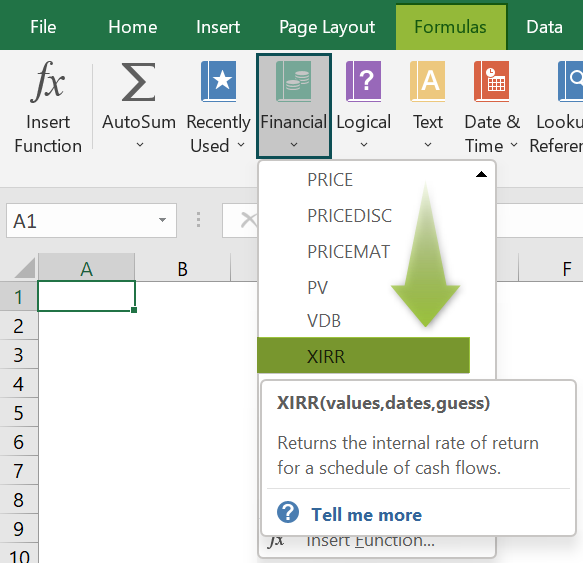# XIRR In ExcelArticle byExcelMojo Team## What Is XIRR Function In Excel?

The Extended Internal Rate of Return or the XIRR function calculates the internal rate of return for an irregular series of cash flows or instalments. This cash flow is not periodic, unlike other payments. The XIRR in Excel is an inbuilt financial function, so we can insert the formula from the “Function Library” or enter it directly in the worksheet.

For example, we apply the XIRR function to calculate the internal rate of return from the given data.

Select cell B2, enter the formula =XIRR(B2:B4,A2:A4), and press the “Enter” key.

The result is “4%”, as shown above.

[Output Observation: The negative amount in cell B2 is the deposit as installment or any payment, and the values in cells B3 and B4 are the returns, which is a positive number. The XIRR is then 4%.]

###### Key Takeaways
• The XIRR in Excel calculates the percentile of the internal return rate.
• Although the dates cell values will be irregular payment, we must ensure the payment dates are always in increasing order.
• To input dates in text format is a risk that excel may misinterpret depending on the date system or date interpretation settings.
• The input for the Dates argument should be cell references that holds the dates or the date values returned from the calculated formulas.

### XIRR() Excel Formula

The syntax of the XIRR in Excel Formula is,

The arguments of the XIRR in Excel Formula are,

• values: It is a mandatory argument. It is the array of values that represent the series of cash flows.
• dates: It is a mandatory argument. It is the irregular, periodic series of dates that correspond to the given array values.
• guess: It is an optional argument. It is an initial guess of what the IRR will be.

### How To Use XIRR Excel Function?

We can use the XIRR in Excel in 2 ways, namely,

1. Access from the Excel ribbon.
2. Enter in the worksheet manually.

#### Method #1 – Access from the Excel ribbon

Choose an empty cell for the output → select the “Formulas” tab → go to the “Function Library” group → click the “Financial” option drop-down → select the “XIRR” function, as shown below.

The “Function Arguments” window appears. Enter the arguments in the “Values”, “Dates”, and “Guess” fields → click “OK”, as shown below.

#### Method #2 – Enter in the worksheet manually

1. Select an empty cell for the output.
2. Type =XIRR( in the selected cell. [Alternatively, type =X and double-click the XIRR function from the list of suggestions shown by Excel.]
3. Enter the arguments as cell values or cell references in excel and close the brackets.
4. Press the “Enter” key.

We will calculate the internal rate of return in Excel using XIRR Function for the date and cash flows.

In the table, the data is,

• Column A contains the Date.
• Column B contains the Cashflow.

The steps to evaluate the cashflows by the XIRR in Excel are,

1. Select cell B7, and enter the formula =XIRR(B2:B6, i.e., the cash flow value.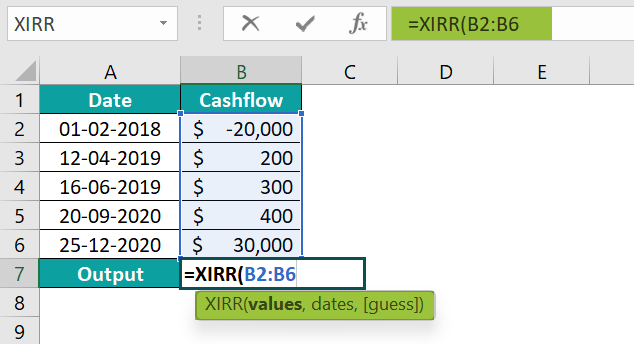2. Enter the value of ‘dates’ as “A2:A6”. The formula is =XIRR(B2:B6,A2:A6,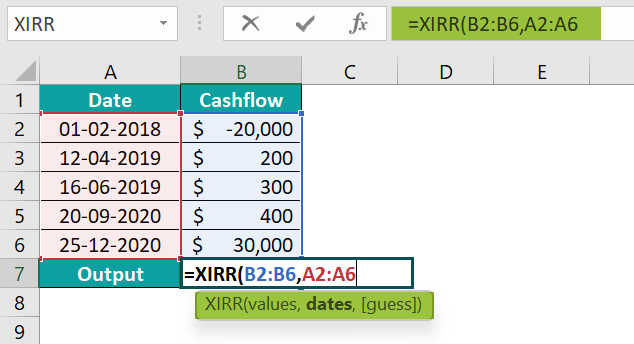3. Enter the value of ‘guess’ as “1”, and close the brackets. The complete formula is =XIRR(B2:B6,A2:A6,1).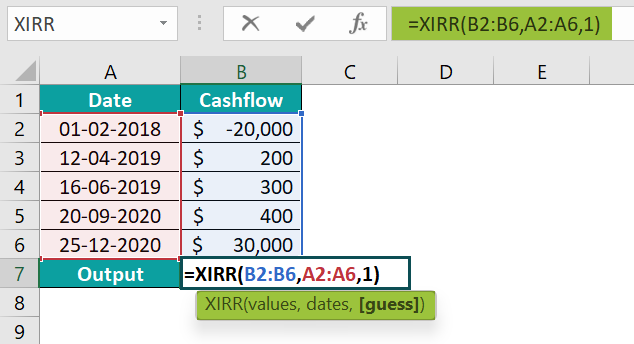4. Press the “Enter” key. The result is “16%”, as shown below.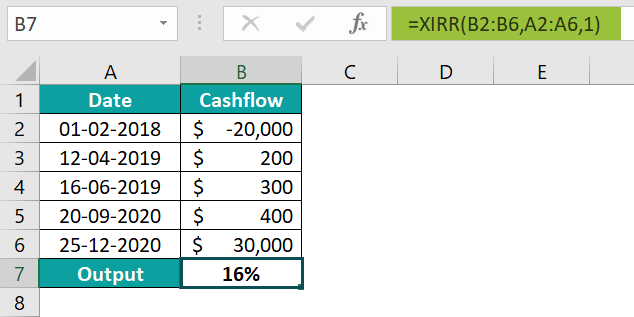### Examples

We will understand some advanced scenarios using the XIRR in Excel examples.

#### Example #1

Mr. Ronald invested in the mutual funds, the image below depicts the date and cash flows, and we will calculate the internal rate of return using the XIRR in Excel.

In the table, the data is,

• Column A contains the Date.
• Column B contains the Cashflow.

The procedure to evaluate the cashflows by the XIRR in Excel is,

Select cell B7, enter the formula =XIRR(B2:B6,A2:A6), and press the “Enter” key.

The result is “61%”, as shown above.

#### Example #2

XYZ company invested in certain bonds in the market, the image below depicts the date and cash flows, and we will calculate the internal rate of return using the XIRR in Excel.

In the table, the data is,

• Column A contains the Date.
• Column B contains the Cashflow.

The procedure to evaluate the cashflows by the XIRR in Excel is,

Select cell B7, enter the formula =XIRR(B2:B6,A2:A6), and press the “Enter” key.

The result is “1%”, as shown above.

#### Example #3

Mrs. Darvi Peterson invested in the funds in the financial market, the image below depicts the date and cash flows, and we will calculate the internal rate of return using the XIRR in Excel.

In the table, the data is,

• Column A contains the Date.
• Column B contains the Cashflow.

The procedure to evaluate the cashflows by the XIRR in Excel is,

Select cell B7, enter the formula =XIRR(B2:B6,A2:A6), and press the “Enter” key.

The result is “41%”, as shown above.

#### Example #4

We will calculate the internal rate of return using the XIRR in Excel, which shows errors due to irregular dates for the date and cash flows.

In the table, the data is,

• Column A contains the Date.
• Column B contains the Cashflow.

The procedure to evaluate the cashflows by the XIRR in Excel is,

Select cell B5, enter the formula =XIRR(B2:B4,A2:A4), and press the “Enter” key.

The result is a “#NUM!” error, as shown above.

### Important Things To Note

• The “#Value!” error occurs if any of the supplied dates aren’t recognized as valid Excel dates.
• The numbers in dates are truncated to integers.
• The “#NUM!” error occurs when:
• The values and dates value arrays are of different lengths.
• The array values don’t have one negative or at least one positive value.
• The calculation converges after 100 iterations.

1. How do I calculate XIRR in Excel?

The XIRR function in Excel is calculated as follows:

1) Select an empty cell for the output.
2) Type =XIRR( in the selected cell. [Alternatively, type =X and double-click the XIRR function from the list of suggestions shown by Excel.]
3) Enter the arguments as cell values or cell references and close the brackets.
4) Press the “Enter” key.

For example, the image depicts the date and cash flows, and we will calculate the internal rate of return using the XIRR in Excel.

In the table, the data is,
Column A contains the Date.
Column B contains the Cashflow.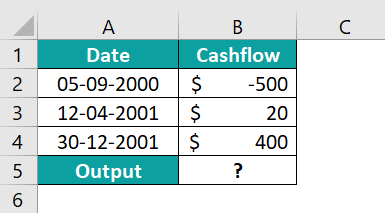The procedure to evaluate the cashflows by the XIRR in Excel are,
Select cell B5, enter the formula =XIRR(B2:B4,A2:A4), and press the “Enter” key.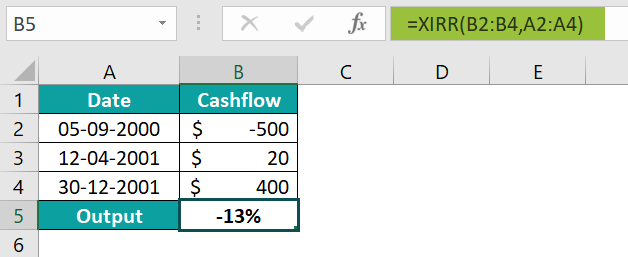The result is “-13%”, as shown above. Therefore, the XIRR calculated is a negative percentile.

2. What is XIRR in Excel?

The XIRR in Excel returns the internal rate of return for continuous cash flows either as investment or instalments that are not periodic, i.e., weekly, monthly, etc.

The syntax of the XIRR function in Excel is =XIRR(values,dates,[guess])

3. Where is the XIRR in Excel Function?

The XIRR in Excel is in the “Formulas” tab, as follows:

Choose an empty cell for the output → select the “Formulas” tab → go to the “Function Library” group → click the “Financial” option drop-down → select the “XIRR” function, as shown below.